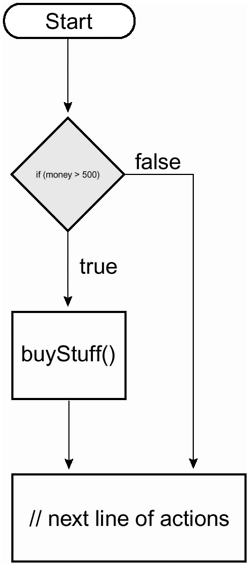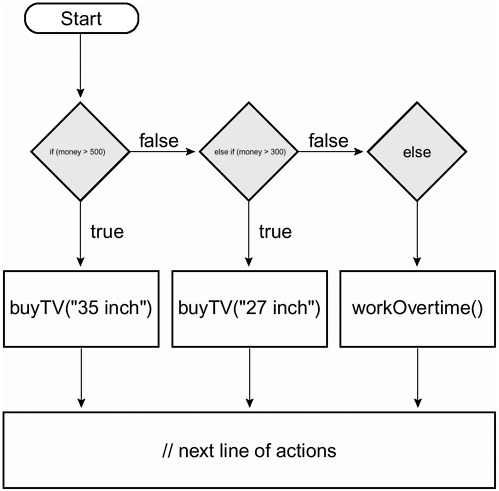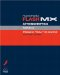# CONTROLLING A SCRIPT S FLOW

### CONTROLLING A SCRIPT'S FLOW

Typically, actions in your scripts execute consecutively, from beginning to end a sequence that represents your script's flow. Using conditional logic, you can control this flow by scripting specific actions to execute only when a specific condition (or condition) is met or exists in your movie. By implementing conditional logic in your scripts, you give your movie the ability to make decisions and take action based on various conditions you've set it up to analyze an ability that allows your movies to take on a life of their own. The following conditional statements, or phrases, are used to implement conditional logic in your scripts.

#### If/Then Statement

At the heart of conditional logic is the simple If/Then statement. Take a look at the following example:

` if (moneySaved > 500) {  buyStuff();  }  // next line of actions… `

In the above script, the buyStuff() function is called only if the variable moneySaved has a value greater than 500. If moneySaved is equal to or less than 500, the buyStuff() function call is ignored and actions immediately below the if statement are executed.At its core, a conditional statement looks at a circumstance (whatever is placed within parentheses) and determines whether that circumstance is true or false . If it is true , actions within the statement are executed; if false, they're ignored. Thus, when creating an if statement, you're essentially stating the following:

` if (…what is shown here is true) {    Do this;  } `

The data you place within parenthesis represents the condition to be analyzed; the data within the curly braces ({}) represents the actions to be taken if the condition exists.

As in real life, sometimes your if statement needs to analyze multiple conditions before taking a single action or set of actions. Take a look at the following example:

` if (moneySaved > 500 && billsPaid == true) {    buyStuff()  } `

In this script the AND operator (&& ) has been added to the statement so that now the buyStuff() function is called only if moneySaved is more than 500 and billsPaid is true . If either condition is false , buyStuff() will not be called. Using the OR operator (|| ) allows you to take a slightly different approach. Take a look at the following example:

` if (moneySaved > 500 || wonLottery == true) {    buyStuff()  } `

In this script, the buyStuff() function is called if either moneySaved has a value greater than 500 or wonLottery has a value of true . Both conditions do not need to be true as is the case when using the AND operator.

You can mix these operators together to create sophisticated conditional statements like the following:

` if (moneySaved > 500 && billsPaid == true || wonLottery == true) {    buyStuff()  } `

In this script the buyStuff() function is called only if moneySaved is more than 500 and billsPaid is true or if wonLottery has a value of true .

Following is a list of the common operators (known as comparison operators because they're used to compare values) used in conditional logic, as well as a brief description and example of how they are used:

OPERATOR

DESCRIPTION

EXAMPLE

==

Checks for equality

if (name == "Derek") . This says, "If name has an exact value of Derek, do the following…"

!=

Checks for inequality

if (name != "Derek") . This says, "If name has a value other than Derek, do the following…"

<

Less than

if (age < 30) . This says, "If age has a value less than 30, do the following…"

>

Greater than

if (age > 30) . This says, "If age has a value greater than 30, do the following…"

<=

Less than or equal to

if (age <= 30) . This says, "If age has a value less than or equal to 30, do the following…"

>=

Greater than or equal to

if (age >= 30) . This says, "If age has a value greater than or equal to 30, do the following…"

&&

Logical AND

if (day == "Friday" && pmTime > 5) . This says, "If day has a value of Friday and pmTime has a value greater than five, do the following…"

||

Logical OR

if (day == "Saturday" day == "Sunday") . This says, "If day has a value of Saturday or Sunday, do the following…"

TIP

A common mistake when checking equality is to insert a single equals sign (=) where a double equals sign (==) belongs. Use a single equals sign to assign a value (for example, money = 300) ; use a double equals sign to check for equality. Thus, money == 300 does not assign a value of 300 to money. Rather, it asks whether money has a value of 300 .

TIP

Although comparing numbers is straightforward after all, most of us understand that 50 is less than 100 comparing text values may be less obvious. "Derek" doesn't equal "derek" even though the same letters are used. With string values, A has a lower value than Z, and lowercase letters have greater values than uppercase letters. Thus, if A has a value of 1, z has a value of 52 (ABCBEFGHIJKLMNOPQRSTUVWXYZabcdefghijklmnopqrstuvwxyz).

#### If/Else If Statement

An if/else if statement is similar to the basic if statement we just discussed; however, it enables your script to react to multiple conditions. Take a look at the following example:

` if (money > 500) {    buyTV("35 inch");  } else if (money > 300) {    buyTV("27 inch");  } `This script executes varying actions depending on the value of money . It says, "If money has a value greater than 500, buy the 35-inch TV." If money has a value less than 500, this part of the script is ignored and the next condition is examined. The next condition says that if money has a value greater than 300, buy the 27-inch TV. Thus, if money has a value of 450 when this script is executed, the first part of the statement is ignored (because 450 is not greater than 500), but the second part of the statement is executed (because a value of 450 is greater than 300). If money had a value of less than 300, both parts of this statement would be ignored.

You can create several lines of if/else if statements that can react to dozens of conditions.

#### If/Else Statement

An if/else statement allows your script to take action if none of the conditions in the statement prove true. Consider this the fail-safe part of the statement.

` if (money > 500) {    buyTV("35 inch");  } else if (money > 300) {    buyTV("27 inch");  } else {    workOvertime ();  } `In this script, if money does not have a value of at least 300, neither of the conditions analyzed will be true , and thus neither of the actions under them will be executed. Instead, the actions under the else part of the statement are executed a bit like saying the following:

` if (…this is true) {    // Do this  } otherwise if (…this is true) {    // Do this  } otherwise, if none of the above conditions are true {    // Do this  } `

#### Conditional Statement Variations

The statements we've discussed up to this point (and the syntax used) form the basis of most conditional logic you'll use in your projects. However, as with most things in ActionScript, there's more than one way to script a conditional statement. Let's take a look at a couple of these variations and how you might use them to shorten your code and make it easier to read. The following variations have no real advantage over the ones we've already discussed; it really comes down to personal preference.

A switch statement which is a variation on the if /else statement can be extremely useful when you want a script to take a specific set of actions when an exact value exists. Take a look at how the following statement works:

` switch (favoriteBand) {  case "Beatles":    gotoAndPlay("Beatles");    break;  case "U2":    gotoAndPlay("U2");    break;  default:    gotoAndPlay("Slim Whitman");  } `

First, in parentheses, there is a single expression (most often a variable), which is evaluated once. The value of the expression is then compared with the values for each case in the structure. If there's a match, the block of code associated with that case is executed. If no match is found, the default statement (at the end) is executed. break is used in each case in order to prevent the code from running into the next case automatically if a match has already been found. What can be checked in each case includes string values (shown), numeric values, and Boolean values of true and false (though for Boolean values, the variation we describe next represents an easier and more appropriate choice).

The ternary operator (?:)lets us write a simple if /else statement in one line. Take, for example, the following:

` myMood = (money > 1000000) ? "Happy" : "Sad"; `

If the condition within the parenthesis evaluates to true , the first expression, "Happy," is set as the value of myMood . If the condition is false , the second expression, "Sad," is used. This single line of ActionScript accomplishes the same thing as the following lines of code:

` if (money > 1000000) {    myMood = "Happy";  } else {    myMood = "Sad";  } `

Here's an example of an extended way to use the ternary operator:

` myMood = "I'm " + (money > 1000000) ? "happy" : "sad"; `

In this example, myMood will have a value of either "I'm happy" or "I'm sad," depending on whether the value of money is greater than or less than 1000000.

The ternary operator is commonly used to toggle variable states. Take, for example, the following:

` playSound = (playSound) ? false : true; `

Every time the above line of ActionScript is executed, the variable playSound is changed from true to false or from false to true .Macromedia Flash MX ActionScripting: Advanced Training from the Source
ISBN: 0201770229
EAN: 2147483647
Year: 2002
Pages: 161